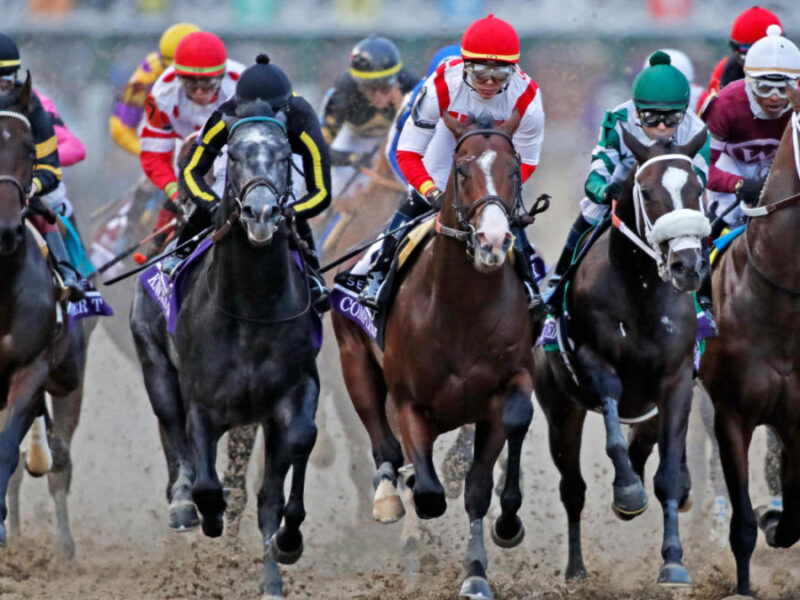# Understanding Different Types of Horse Racing Odds

Racing odds can be pretty intimidating for a beginner. If you are new to horse race betting all the numbers will leave you confused.

The numbers and figures you see are nothing but horse racing odds. You need to use the odds to place bets.

One of the most important qualities of a punter is to read the odds. You need to know how to read and understand the odds.

However, before you learn how to read the racing odds, you need to learn about the different types of odds.

Types of Racing Odds:

When it comes to betting, odds play a very important role. So you need to know how to read the odds. As there are different type of odds, you need to learn about it first. Here are the types of odds in horse race betting.

Fractional Betting Odds: As the name goes, these bets are in the form of a fraction. In the UK and other European countries, bookies and punters prefer using fractional odds. The odds will always be in a fraction. There will be two numbers. The first number will be divided by the second number. For example, if the odds are 3/1, you need to divide three by 1 to get the odds in the decimal form. The answer will be three, which mean the odds are 3-1. So if you put a stake of \$5 you will get \$5 X 3 =\$15 in return. Apart from earning a profit, you will also get the stake money back, i.e. \$5. This is how fractional odds work. If the odds are 16/2, you have to divide 16 by two, which become 8. So if you put a wager of \$1, you will get a profit of \$8.

• Decimal Betting Odds:

This type of odds is in the form of a decimal number. It is not really common amongst bookies. Only a few bookies use this type of odds. However, decimal odds are easier than fractional odds. You don’t have to divide any number. Instead, you can directly multiply the odds with the stake to calculate the profit. For example, a bet of \$100 at 3.0 odds will give you a return of \$300. You need to subtract one from the decimal odd and then multiply it with the stake. After that, you need to add the stake money back. So 3.0-1 = 2.0. So if you multiply \$100 by 2.0 = \$200. Now you need to add the stake money, i.e. \$100.

Probability of Winning:

Once you understand the type of odds, you need to understand the probability of winning. When it comes to racing odds, you need to check the odds of the favoured horse. It will always be low so that even if people put money at stake, they will make less profit. On the other hand, an underdog horse will have higher odds so that people can win more profit by putting less money at stake.

These are the two popular types of racing odds. Make sure you check the winning probability of the odds before placing bets.

### Related Posts### Food Plot: Forage to Attract Wildlife### Exploring the Environmental Benefits of Vermicomposting### Swimming with dolphins in Punta Cana Oceans Adventure Park